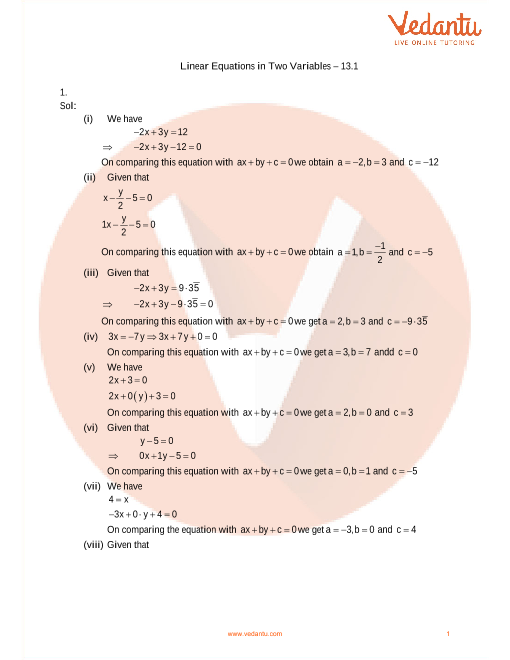RD Sharma Class 9 Solutions Chapter 13 - Linear Equation In Two Variables (Ex 13.1) Exercise 13.1

RD Sharma Class 9 Solutions Chapter 13 - Linear Equation In Two Variables (Ex 13.1) Exercise 13.1 - Free PDF

Free PDF download of RD Sharma Class 9 Solutions Chapter 13 - Linear Equation In Two Variables Exercise 13.1 solved by Expert Mathematics Teachers on Vedantu.com. All Chapter 13 - Linear Equation In Two Variables Ex 13.1 Questions with Solutions for RD Sharma Class 9 Maths to help you to revise complete Syllabus and Score More marks. Register for online coaching for IIT JEE (Mains & Advanced) and other Engineering entrance exams.